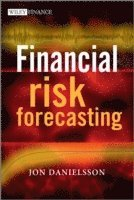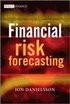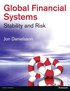Fler böcker inom
Format
Inbunden (Hardback)
Språk
Engelska
Antal sidor
294
Utgivningsdatum
2011-03-25
Upplaga
1
Förlag
John Wiley & Sons Inc
Illustrationer
black & white illustrations, black & white tables, figures
Dimensioner
254 x 177 x 25 mm
Vikt
657 g
Antal komponenter
1
Komponenter
1669:Standard B&W 6.69 x 9.61 in or 244 x 170 mm (Pinched Crown) Case Laminate on White w/Gloss Lam
ISBN
9780470669433

### Du kanske gillar# Financial Risk Forecasting

The Theory and Practice of Forecasting Market Risk with Implementation in R and Matlab

Inbunden Engelska, 2011-03-25
549
Skickas inom 7-10 vardagar.
Fri frakt inom Sverige för privatpersoner.
Boken kan tyvärr inte levereras innan julafton.
Finns även som
809
809
Financial Risk Forecasting is a complete introduction to practical quantitative risk management, with a focus on market risk. Derived from the authors teaching notes and years spent training practitioners in risk management techniques, it brings together the three key disciplines of finance, statistics and modeling (programming), to provide a thorough grounding in risk management techniques. Written by renowned risk expert Jon Danielsson, the book begins with an introduction to financial markets and market prices, volatility clusters, fat tails and nonlinear dependence. It then goes on to present volatility forecasting with both univatiate and multivatiate methods, discussing the various methods used by industry, with a special focus on the GARCH family of models. The evaluation of the quality of forecasts is discussed in detail. Next, the main concepts in risk and models to forecast risk are discussed, especially volatility, value-at-risk and expected shortfall. The focus is both on risk in basic assets such as stocks and foreign exchange, but also calculations of risk in bonds and options, with analytical methods such as delta-normal VaR and duration-normal VaR and Monte Carlo simulation. The book then moves on to the evaluation of risk models with methods like backtesting, followed by a discussion on stress testing. The book concludes by focussing on the forecasting of risk in very large and uncommon events with extreme value theory and considering the underlying assumptions behind almost every risk model in practical use that risk is exogenous and what happens when those assumptions are violated. Every method presented brings together theoretical discussion and derivation of key equations and a discussion of issues in practical implementation. Each method is implemented in both MATLAB and R, two of the most commonly used mathematical programming languages for risk forecasting with which the reader can implement the models illustrated in the book. The book includes four appendices. The first introduces basic concepts in statistics and financial time series referred to throughout the book. The second and third introduce R and MATLAB, providing a discussion of the basic implementation of the software packages. And the final looks at the concept of maximum likelihood, especially issues in implementation and testing. The book is accompanied by a website - www.financialriskforecasting.com which features downloadable code as used in the book.

## Passar bra ihop

1.2. +
3.De som köpt den här boken har ofta också köpt Global Financial Systems av Jon Danielsson (häftad).

## Kundrecensioner

Har du läst boken? Sätt ditt betyg »

## Övrig information

Jon Danielsson has a PhD in the economics of financial markets and is a reader in finance at the London School of Economics. His research interests include financial stability, extreme market movements, risk, market liquidity and financial crisis. He has published extensively in both academic and practitioner journals, has consulted with a variety of private sector and public institutions, frequently gives executive education courses and has presented his work in a number of universities and institutions. In addition, he has been a frequent commentator of issues in financial markets in the media, appearing on CNN, the BBC, and many other TV and radio stations, with comments and op-ed pieces in newspapers like the Financial Times.

## Innehållsförteckning

Preface. Acknowledgments. Abbreviations. Notation. 1 Financial markets, prices and risk. 1.2 S&P 500 returns. 1.3 The stylized facts of financial returns. 1.4 Volatility. 1.5 Nonnormality and fat tails. 1.6 Identification of fat tails. 1.7 Nonlinear dependence. 1.8 Copulas. 1.9 Summary. 2 Univariate volatility modeling. 2.1 Modeling volatility. 2.2 Simple volatility models. 2.3 GARCH and conditional volatility. 2.4 Maximum likelihood estimation of volatility models. 2.5 Diagnosing volatility models. 2.6 Application of ARCH and GARCH. 2.7 Other GARCH-type models. 2.8 Alternative volatility models. 2.9 Summary. 3 Multivariate volatility models. 3.1 Multivariate volatility forecasting. 3.2 EWMA. 3.3 Orthogonal GARCH. 3.4 CCC and DCC models. 3.5 Estimation comparison. 3.6 Multivariate extensions of GARCH. 3.7 Summary. 4 Risk measures. 4.1 Defining and measuring risk. 4.2 Volatility. 4.3 Value-at-risk. 4.4 Issues in applying VaR. 4.5 Expected shortfall. 4.6 Holding periods, scaling and the square root of time. 4.7 Summary. 5 Implementing risk forecasts. 5.1 Application. 5.2 Historical simulation. 5.3 Risk measures and parametric methods. 5.4 What about expected returns? 5.5 VaR with time-dependent volatility. 5.6 Summary. 6 Analytical value-at-risk for options and bonds. 6.1 Bonds. 6.2 Options. 6.3 Summary. 7 Simulation methods for VaR for options and bonds. 7.1 Pseudo random number generators. 7.2 Simulation pricing. 7.3 Simulation of VaR for one asset. 7.4 Simulation of portfolio VaR. 7.5 Issues in simulation estimation. 7.6 Summary. 8 Backtesting and stress testing. 8.1 Backtesting. 8.2 Backtesting the S&P 500. 8.3 Significance of backtests. 8.4 Expected shortfall backtesting. 8.5 Problems with backtesting. 8.6 Stress testing. 8.7 Summary. 9 Extreme value theory. 9.1 Extreme value theory. 9.2 Asset returns and fat tails. 9.3 Applying EVT. 9.4 Aggregation and convolution. 9.5 Time dependence. 9.6 Summary. 10 Endogenous risk. 10.1 The Millennium Bridge. 10.2 Implications for financial risk management. 10.3 Endogenous market prices. 10.4 Dual role of prices. 10.5 Summary. APPENDICES. A Financial time series. A.1 Random variables and probability density functions. A.2 Expectations and variance. A.3 Higher order moments. A.4 Examples of distributions. A.5 Basic time series concepts. A.6 Simple time series models. A.7 Statistical hypothesis testing. B An introduction to R. B.1 Inputting data. B.2 Simple operations. B.3 Distributions. B.4 Time series. B.5 Writing functions in R. B.6 Maximum likelihood estimation. B.7 Graphics. C An introduction to Matlab. C.1 Inputting data. C.2 Simple operations. C.3 Distributions. C.3.1 Normality tests. C.4 Time series. C.5 Basic programming and M-files. C.6 Maximum likelihood. C.7 Graphics. D Maximum likelihood. D.1 Likelihood functions. D.2 Optimizers. D.3 Issues in ML estimation. D.4 Information matrix. D.5 Properties of maximum likelihood estimators. D.6 Optimal testing procedures. Bibliography. Index.